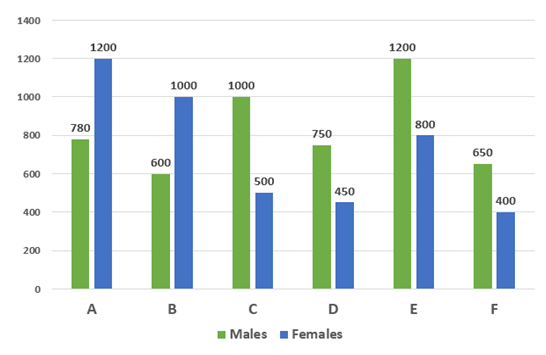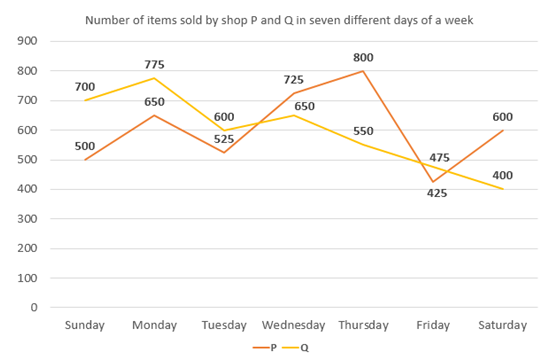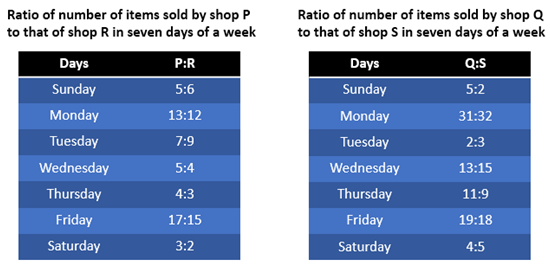# Quantitative Aptitude Questions (Data Interpretation) for RRB PO Prelims 2018 Day-161

Dear Readers, IBPS RRB is conducting Online Examination for the recruitment of PO. To enrich your preparation here we have providing new series of Data Interpretation – Quantitative Aptitude Questions. Candidates those who are appearing in RRB PO Prelims Exams can practice these Quantitative Aptitude average questions daily and make your preparation effective.

Click “Start Quiz” to attend these Questions and view Solutions

Directions (1 – 5): Study the following information carefully and answer the questions given questions.

The following bar graph represents number of males and females working in six different companies.1) Number of males in company A is approximately what percent more

than the number of females in company D?

a) 60 %

b) 73 %

c) 50 %

d) 80 %

e) 65 %

2) Find the difference between total number of males and total number of females in all the companies together?

a) 590

b) 650

c) 710

d) 630

e) None of these

3) Find the ratio of total number of females working in company A, B and C together and the total number of males working in company D, E and F together.

a) 26: 25

b) 27: 26

c) 25: 24

d) 24: 23

e) None of these

4) Total number of males of company B, C and E together is approximately what percent of total number of females of company A, D and F together?

a) 112 %

b) 154 %

c) 136 %

d) 95 %

e) 168 %

5) Find the average number of female employees in all the given companies together?

a) 650

b) 725

c) 800

d) 575

e) None of these

Directions (6 – 10): Study the following information carefully and answer the questions given below:6) Total number of items sold by shop P in all the given days together is approximately what percent of the total number of items sold by shop Q in all the given days together?

a) 110 %

b) 100 %

c) 85 %

d) 120 %

e) 135 %

7) The number of items sold by shop R in Wednesday and Thursday together is approximately what percent more than the number of items sold by shop S in Thursday and Friday together?

a) 20 %

b) 40 %

c) 30 %

d) 10 %

e) 45 %

8) Find the ratio between the number of items sold by shop R in Sunday, Monday and Tuesday together to that of the number of items sold by shop S in Wednesday, Thursday and Friday together?

a) 23: 21

b) 21: 23

c) 22: 25

d) 25: 22

e) None of these

9) Find the total number of items sold by shop R in all the given days together?

a) 3083

b) 3830

c) 3803

d) 3038

e) None of these

10) Find the difference between total number of items sold by shop Q to that of shop S in all the given days together?

a) 45

b) 29

c) 20

d) 30

e) None of these

Required % = [(780 – 450)/450]*100 = 73 %

Total number of males = 780 + 600 + 1000 + 750 + 1200 + 650 = 4980

Total number of females = 1200 + 1000 + 500 + 450 + 800 + 400 = 4350

Required difference = 4980 – 4350 = 630

Total number of females working in company A, B and C together

= > 1200 + 1000 + 500 = 2700

Total number of males working in company D, E and F together

= > 750 + 1200 + 650 = 2600

Required ratio = 2700: 2600 = 27: 26

Total number of males of company B, C and E together

= > 600 + 1000 + 1200 = 2800

Total number of females of company A, D and F together

= > 1200 + 450 + 400 = 2050

Required % = (2800/2050)*100 = 136 %

Total number of females in all the given companies together

= > 1200 + 1000 + 500 + 450 + 800 + 400 = 4350

Required average = 4350/6 = 725

Total number of items sold by P all the given days together

= > 500 + 650 + 525 + 725 + 800 + 425 + 600 = 4225

Total number of items sold by Q all the given days together

= > 700 + 775 + 600 + 650 + 550 + 475 + 400 = 4150

Required percentage = (4225/4150)*100 = 101.8 % = 100 %

The number of items sold by shop R in Wednesday and Thursday together

= (4/5)*725 + (3/4)*800

= 580 + 600 = 1180

The number of items sold by shop S in Thursday and Friday together

= (9/11)*550 + (18/19)*475

= 450 + 450 = 900

Required percentage = [(1180 – 900)/900]*100 = 31 % = 30 %

The number of items sold by shop R in Sunday, Monday and Tuesday together

= (6/5)*500 + (12/13)*650 + (9/7)*525

= 600 + 600 + 675 = 1875

The number of items sold by shop S in Wednesday, Thursday and Friday together

= (15/13)*650 + (9/11)*550 + (18/19)*475

= 750 + 450 + 450 = 1650

Required ratio = 1875: 1650 = 25: 22

Total number of items sold by shop R all the given days together

= > (6/5)*500 + (12/13)*650 + (9/7)*525 + (4/5)*725 + (3/4)*800 + (15/17)*425 + (2/3)*600

= > 600 + 600 + 675 + 580 + 600 + 375 + 400

= > 3830

Total number of items sold by Q in all the given days together

= > 700 + 775 + 600 + 650 + 550 + 475 + 400 = 4150

Total number of items sold by S in all the given days together

= > (2/5)*700 + (32/31)*775 + (3/2)*600 + (15/13)*650 + (9/11)*550 + (18/19)*475 + (5/4)*400

= > 280 + 800 + 900 + 750 + 450 + 450 + 500

= > 4130

Required difference = 4150 – 4130 = 20